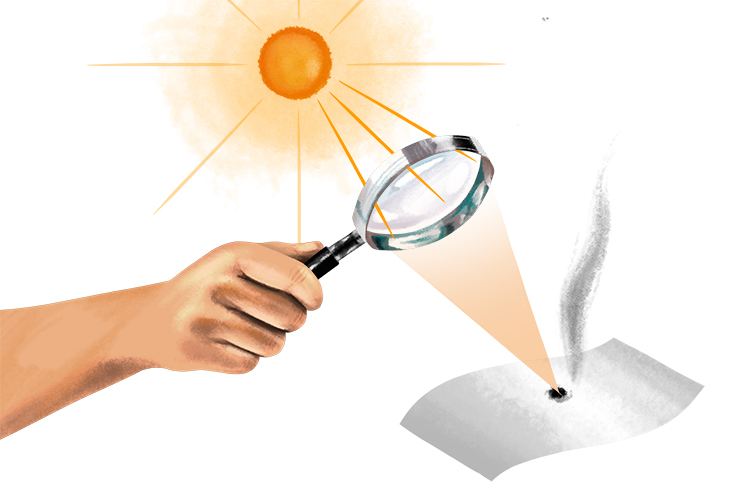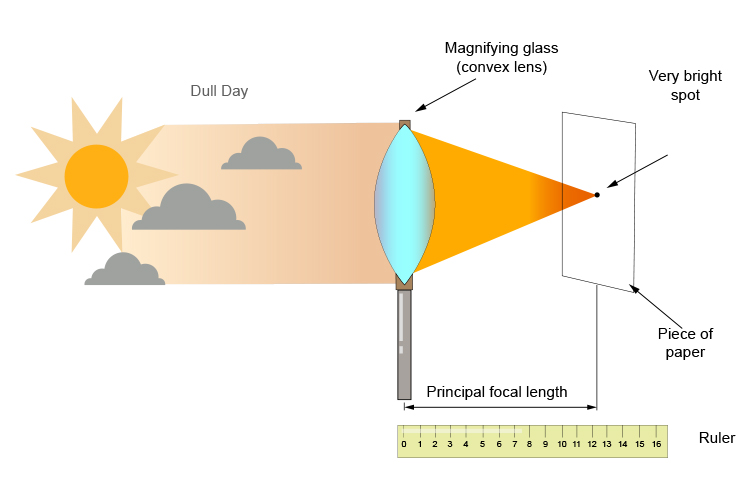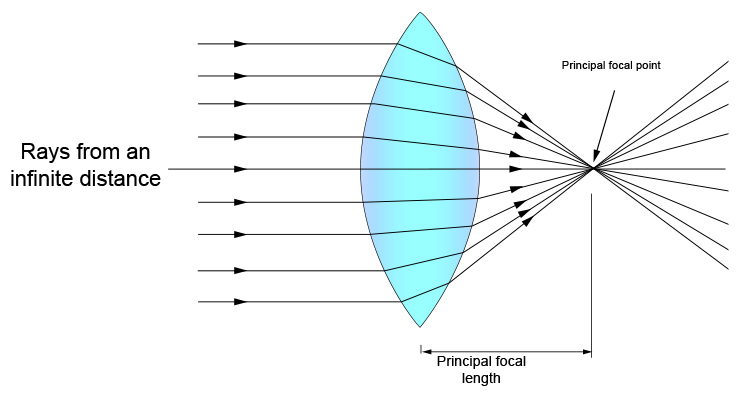# Practical ways to find the principal focal length – magnifying glasses

There are many uses of a convex lens but one use is to use a convex lens to start a fire. Convex lenses used in this way are called magnifying glasses.Alternatively, on a dull day try focusing this light onto a piece of paper and find the brightest point (without setting fire to the paper).

If you measure the distance between the magnifying glass (convex lens) and the piece of paper this will provide you with a rough principal focal length.In ray diagram format this would be: